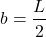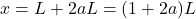# Beam Deflections Jun 2022

Hand calculate beam deflections
I thought I should reassess and resharpen my basic structural engineering skills.

I present a few problems

(a) a concentrated load P at the free end,
(b) a moment M at the free end, and
(c) a uniform load w over the entire beam.
The span is L.
For each loading, determine the beam deflection at the free end of the span.See calculation of cantilever deflections

2. Given a simple beam with a P load at midspan.
The span is L.
Determine the beam rotation at the right end of the span.I solved this problem using the virtual work method.3. Given a simple beam with a P load at at a distance a from the left support.
The span is L; therefore,.
Determine the beam rotation at the right end of the span.
Determine also the deflection for all points x located left of the P loading.I solved this problem using the conjugate beam method (*).Note that ifthen the third equation is equal to the second equation.

4. Given a beam (or plate) with uniform load, w, subjected to a 2 point symmetric lift
The center span, L, is to be determined
The value, a, is a ratio to be determined
The left overhang = the right overhang =Determine the span and the overhang that minimizes the absolute value of the moment along the span
Determine the resulting deflection at the right end of the beam, atAnswer: see hand calculation of deflection given minimized moment
Answer: see calculation of deflection given minimized moment using sympy.physics.continuum_mechanics.beam.Beam (**)
Note that my calculation of a agrees with the well-known value of a.I derived two deflection equations:I determined a few interesting values of a and charted the resulting deflections.

http://khoitsmahq.firstcloudit.com/images%2Ftable_chart.png

http://khoitsmahq.firstcloudit.com/images%2Fjust_chart.png

5. Given a pin-supported bent with uniform EI:The beam span is L.
The column length is μL.
Determine the horizontal deflection of the frame at point B.See calculation of frame deflection

Bonus Material

For the first 2 problems, I checked some algebra using Jupyter, Plotly, Python, and Sympy.

http://khoitsmahq.firstcloudit.com/images%2Fbeam_hand_calc_check.html

Quite a surprise — I discovered a Sympy package named sympy.physics.continuum_mechanics.beam.Beam (**) and checked some of my results using that package too. Note Sympy’s use of singularity functions in cells 4 and 5. I use singularity functions in cells 6 through 10.

http://khoitsmahq.firstcloudit.com/images%2Fshear_and_moment.html

def f_rotation(x, E, I):
sf = SingularityFunction
return (-10*sf(x, 0, 2)/3 +
5*sf(x, 48, 2) -
5*sf(x, 144, 2)/3 +
12800)/(E*I)

def f_deflection(x, E, I):
sf = SingularityFunction
return (12800*x -
10*sf(x, 0, 3)/9 +
5*sf(x, 48, 3)/3 -
5*sf(x, 144, 3)/9)/(E*I)



References